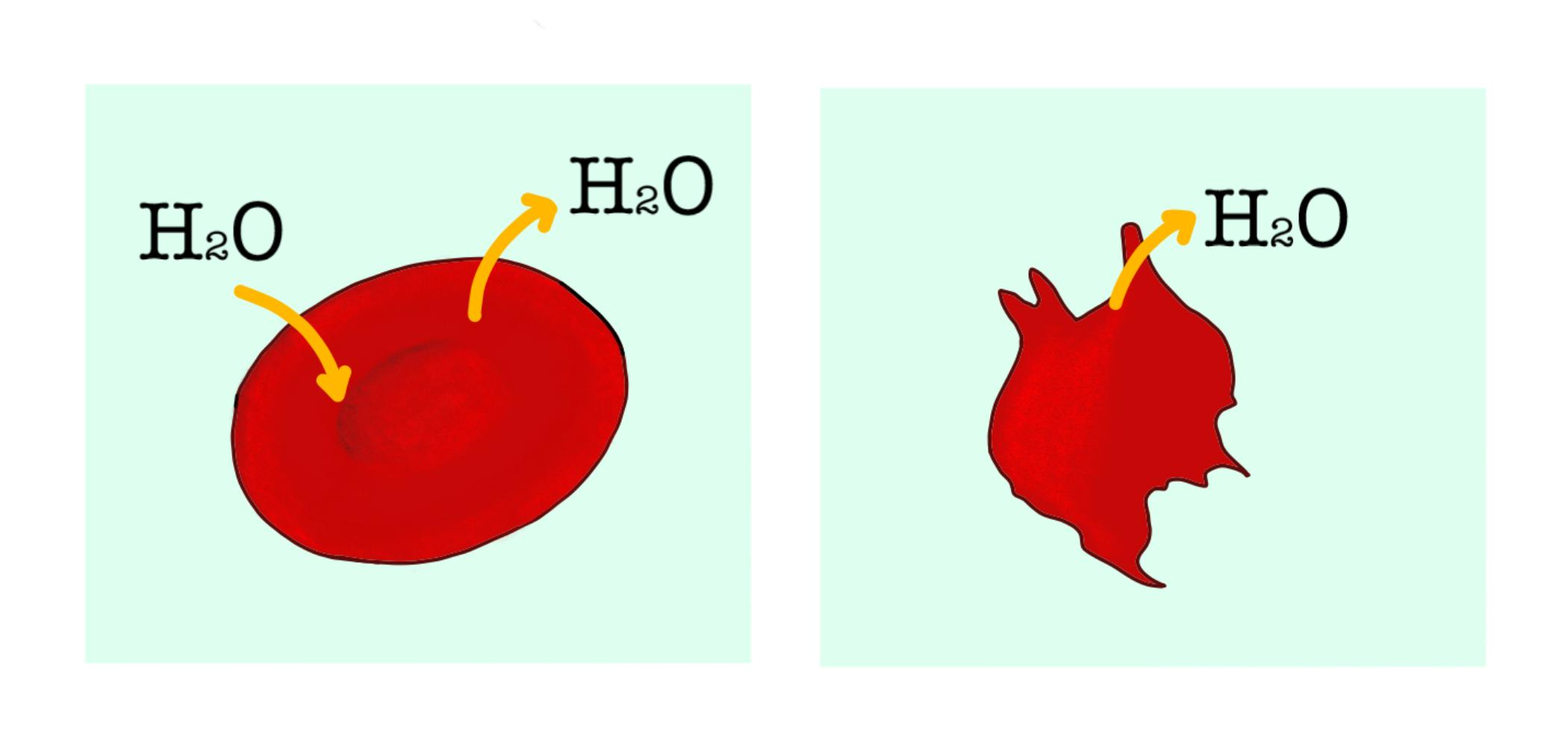# Observe the given figure and answer the question. Explain what has happened to the cell. Consider the cells as A and B respectively.Verified
180.3k+ views
Hint: As seen in the figure A, the ${ H }_{ 2 }O$ molecule is seen going in and out of the red blood cell, in its intact shape. In figure B, ${ H }_{ 2 }O$ is seen leaving the cell and the cell shape looks abnormal. Hence, the entry and exit of water from the cell is a phenomenon that explains the change in the cell shape.